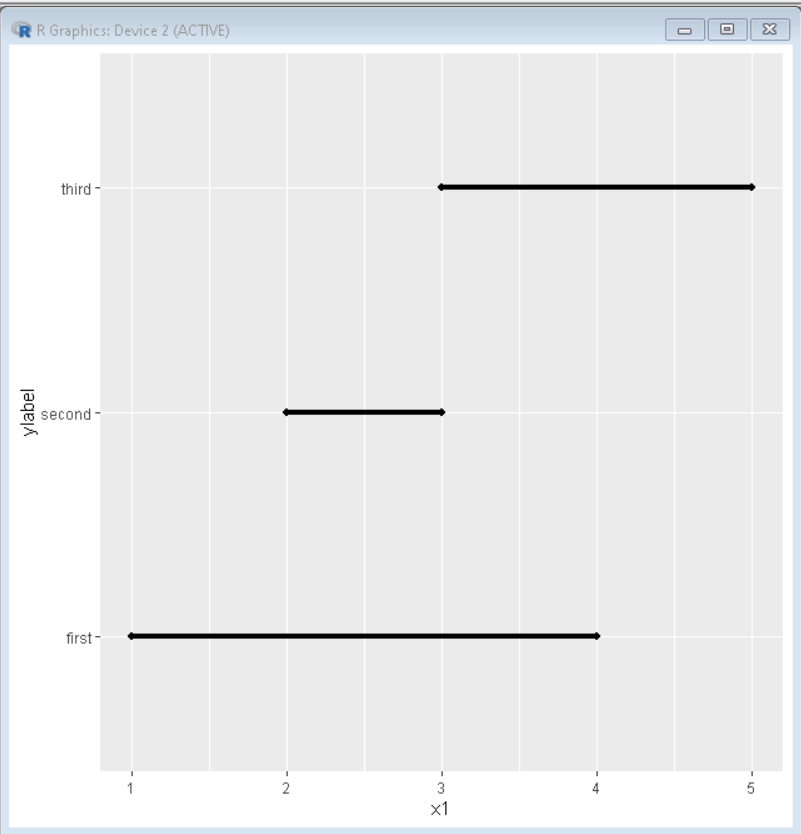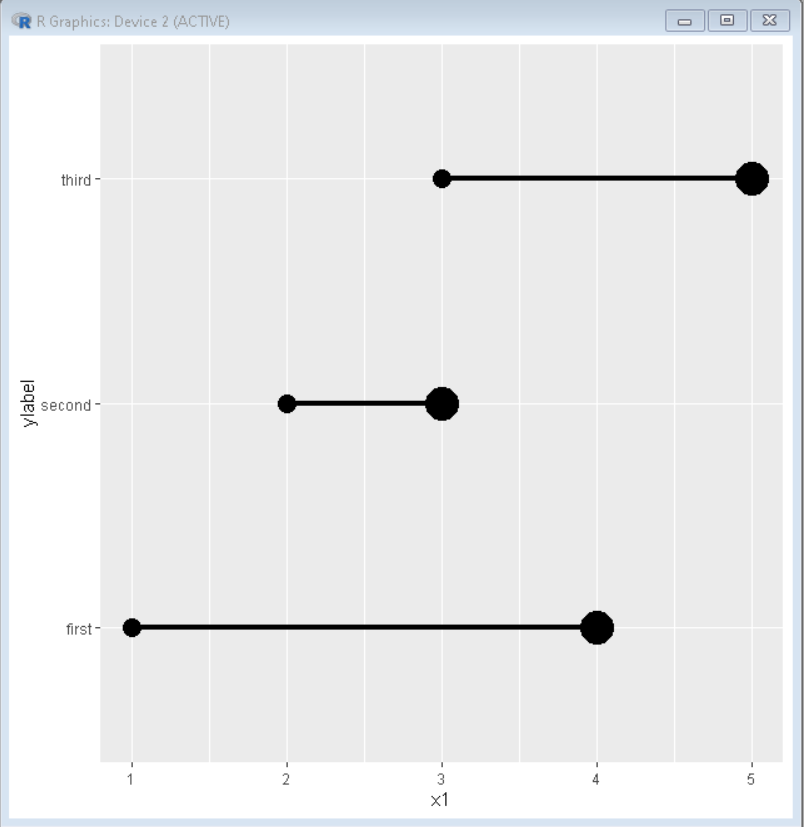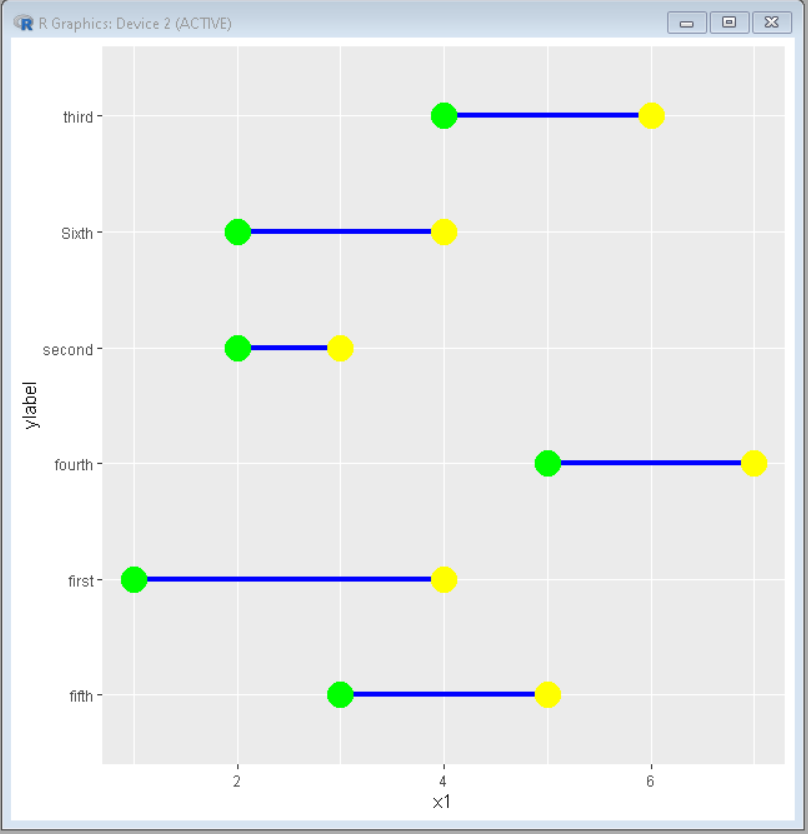Open in App
Not now

# How To Make Dumbbell Plot in R with ggplot2?

• Difficulty Level : Basic
• Last Updated : 23 Aug, 2021

The Dumbbell Plot shows the change between two points in our dataset. It is named so because of its Dumbbell shape. It helps us to understand the span of data categorically. To make Dumbbell Plot in R using ggplot2,  we use the geom_dumbbell() function.

Syntax: geom_dumbbell(data, aes(y, x, xend), size, color, size_x, size_xend, colour_x, colour_xend)

Parameters:

• data: Data frame for plotting data
• size: Size of bar in dumbbell
• color: Color of bar in dumbbell
• size_x: Size of first circle in plot
• colour_x: Colour of first circle in plot
• size_xend: Size of second circle in plot
• colour_xend: Colour of second circle in plot

With ggplot2, Dumbbell plots can be built using geom_dumbbell() function. At least three variables must be provided to aes() that are y, x, and xend.

## Creating basic dumbbell plot

Here is a basic dumbbell plot using the ggplot2 package.

## R

 `# create data sets``ylabel <- ``c``(``"first"``,``"second"``,``"third"``)``x1 <- ``c``(1,2,3)``x2 <- ``c``(4,3,5)``datamain <- ``data.frame``(ylabel,x1,x2)`` ` `# import ggplot2, ggalt and tidyverse``library``(ggplot2) ``library``(ggalt)   ``library``(tidyverse)`` ` `# Draw dumbbell plot``ggplot``() + ``geom_dumbbell``(data = datamain, ``                         ``aes``(y = ylabel,``                             ``x = x1, ``                             ``xend = x2),``                         ``size = 1.5)`

Output:## Customizing Size in Dumbbell Plot:

We can customize the dumbbell plot by changing the radius of the circle in the dumbbell plot or the width of the segment between.

## R

 `# create data sets``ylabel <- ``c``(``"first"``,``"second"``,``"third"``)``x1 <- ``c``(1,2,3)``x2 <- ``c``(4,3,5)``datamain <- ``data.frame``(ylabel,x1,x2)`` ` `# import ggplot2, ggalt and tidyverse``library``(ggplot2) ``library``(ggalt)   ``library``(tidyverse)`` ` `# Draw dumbbell plot``ggplot``() + ``geom_dumbbell``(data = datamain,``                         ``aes``(y = ylabel,``                             ``x = x1,``                             ``xend = x2),``                         ``size = 1.5,``                         ``size_x = 5, ``                         ``size_xend = 9)`

Output:## Customizing Color in Dumbbell Plot:

We can customize color in the dumbbell plot by using color_x and color_xend properties.

## R

 `# create data sets``ylabel <- ``c``(``"first"``,``"second"``,``"third"``,``            ``"fourth"``,``"fifth"``,``"Sixth"``)``x1 <- ``c``(1,2,4,5,3,2)``x2 <- ``c``(4,3,6,7,5,4)`` ` `datamain <- ``data.frame``(ylabel,x1,x2)`` ` `# import ggplot2, ggalt and tidyverse``library``(ggplot2)  ``library``(ggalt)    ``library``(tidyverse)`` ` `# Draw dumbbell plot``ggplot``() +`` ``geom_dumbbell``(data = datamain, ``aes``(y = ylabel,``                                    ``x = x1, ``                                    ``xend = x2),``               ``size = 1.5, color = ``"blue"``, size_x = 7,``               ``size_xend = 7, colour_x = ``"green"``,``               ``colour_xend = ``"yellow"``)`

Output:My Personal Notes arrow_drop_up